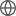Old Web
English
Using radial velocity measurements from four instruments, we report the mass and density of a $2.043\pm0.069 ~\rm{R}_{\oplus}$ sub-Neptune orbiting the quiet K-dwarf Wolf 503 (HIP 67285). In addition, we present improved orbital and transit parameters by analyzing previously unused short-cadence $K2$ campaign 17 photometry and conduct a joint radial velocity-transit fit to constrain the eccentricity at $0.41\pm0.05$. The addition of a transit observation by $Spitzer$ also allows us to refine the orbital ephemeris in anticipation of further follow-up. Our mass determination, $6.26^{+0.69}_{-0.70}~\rm{M}_{\odot}$, in combination with the updated radius measurements, gives Wolf 503 b a bulk density of $\rho = 2.92\pm ^{+0.50}_{-0.44}$ $\rm{g}~\rm{cm}^{-3}$. Using interior composition models, we find this density is consistent with an Earth-like core with either a substantial $\rm{H}_2\rm{O}$ mass fraction ($45^{+19.12}_{-16.15}\%$) or a modest H/He envelope ($0.5\pm0.28\%$). The low H/He mass fraction, along with the old age of Wolf 503 ($11\pm2$ Gyrs), makes this sub-Neptune an opportune subject for testing theories of XUV-driven mass loss while the brightness of its host ($J=8.3$ mag) makes it an attractive target for transmission spectroscopy.